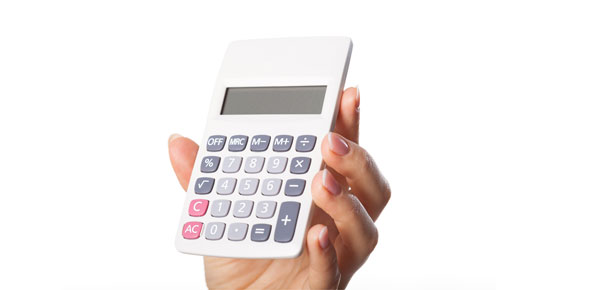# Calculating Molar Mass Grade 9a

5 Questions | Attempts: 194SettingsHow is the molar mass of a substance determined?

• 1.
What is the molar mass of Carbon?
• A.

6 g/mol

• B.

12.01 g/mol

• C.

19.00 g/mol

• D.

1.01 g/mol

• 2.
What is the molar mass of CH4 (methane)?
• A.

12.01 g/mol

• B.

13.02 g/mol

• C.

16.05 g/mol

• D.

32.10 g/mol

• 3.
What is the molar mass of (NH4)2CO3 (Ammonium carbonate)?
• A.

96.11 g/mol

• B.

82.10 g/mol

• C.

106.11 g/mol

• D.

43.01 g/mol

• 4.
What is the molecular mass of Cu3(PO4)2?
• A.

253.49 g/mol

• B.

380.59 g/mol

• C.

110.22 g/mol

• D.

348.59 g/mol

• 5.
What is the molecular mass of Aluminum nitrate Al(NO3)3 from this equation: 2Al(s)   + 3Fe(NO3)2(aq)   à 3Fe(s)  + 2Al(NO3)3(aq)
• A.

213.01 g/mol

• B.

335.98 g/mol

• C.

56.99 g/mol

• D.

141.01 g/mol

## Related TopicsBack to top
×

Wait!
Here's an interesting quiz for you.